# Newton-Raphson iteration for SDOF and MDOF systems

Although the category of nonlinear analysis contains a wide range of different types of analysis, this article is focusing on the force controlled nonlinear analysis. by giving visual explanations of the theoretical background that we applied for the implementation of the analysis procedure. AxisVM uses the Newton-Raphson iteration method to solve the nonlinear problems.

# Newton-Raphson iteration for SDOF system#

The mathematical model of a Single Degree Of Freedom nonlinear system is a nonlinear algebraic equation. It describes the force response of the model with respect to any arbitrary displacement. For a mechanical representation, it can be considered as a simplified model of a bolted joint.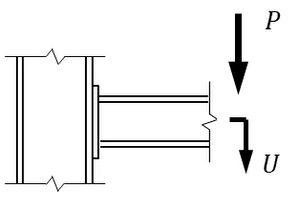We would like to find the displacement solution for a given load P with the Newton-Raphson numerical method. This method contains 2 steps.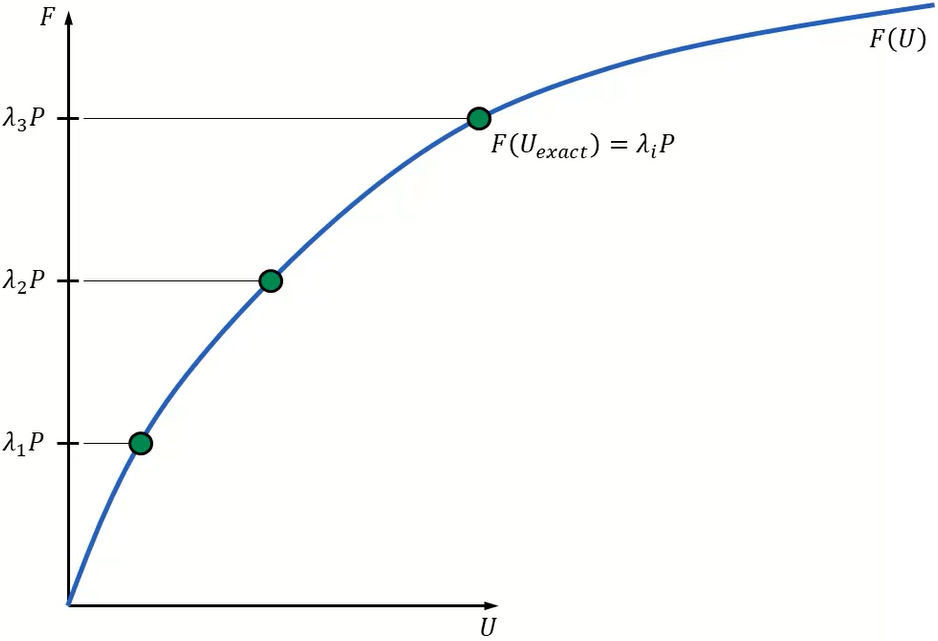Then, an iteration method tires to find the way from the equilibrium point of the last load level to the next one.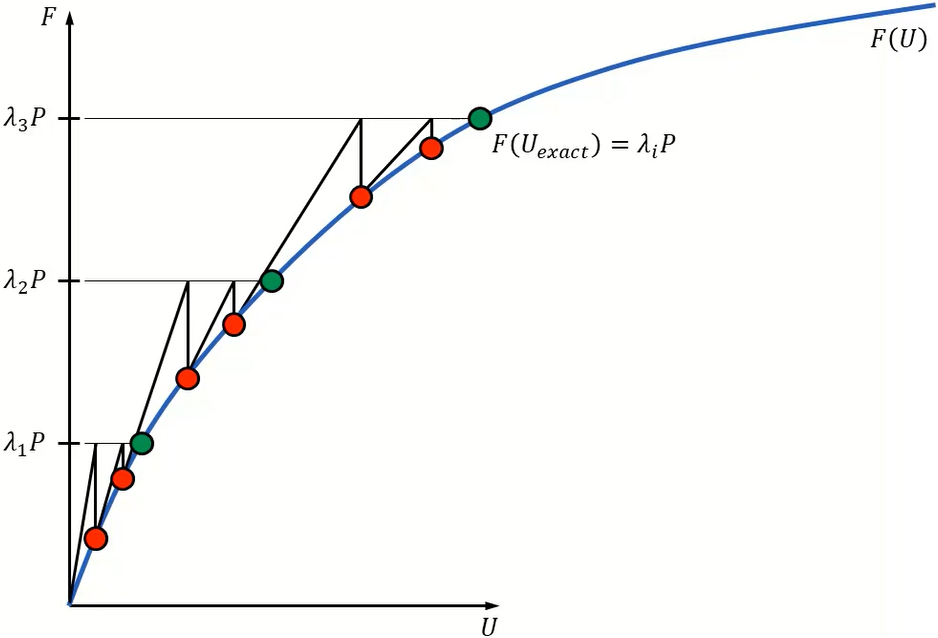The exact solutions at certain load levels are marked by the green circles, while the red circles denote the trial points of the iterations. During an iteration, the nonlinear function is approximated with its tangent.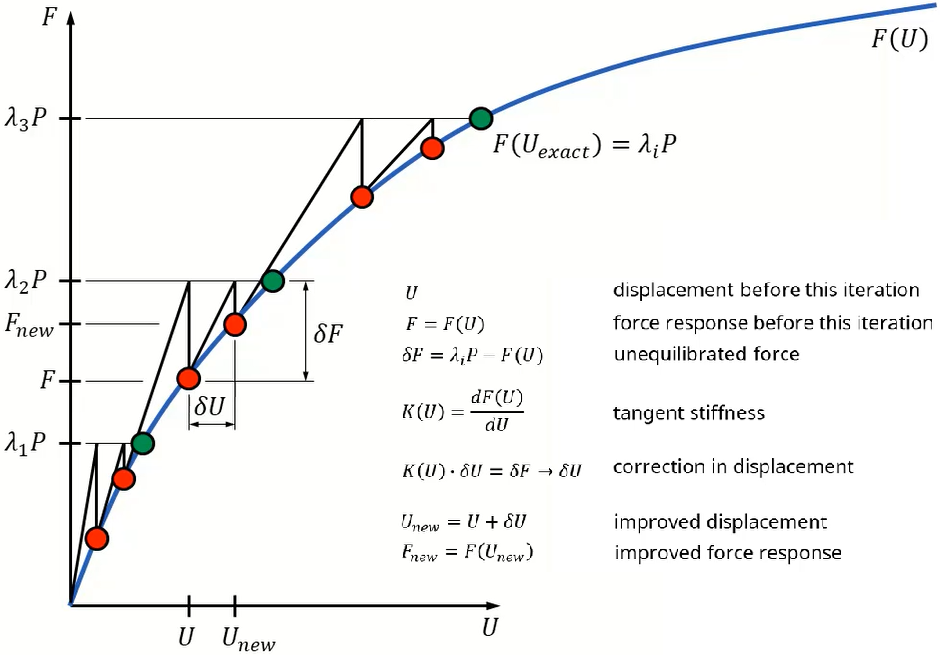The written equations describes an iteration step. The exact solutions can only be approximated. For the decision whether the trial point may be close enough to the exact solution, the fulfilment of predefined convergence criteria is checked.

# Newton-Raphson iteration for MDOF system#

Lets see the Multiple Degree Of Freedom – MDOF case. A simple solution is to change to vector variables with a system of equations. But in this approach a 4 dimensional diagram would be necessary to visualize the 2 displacement and the 2 force components of a 2 DOF system.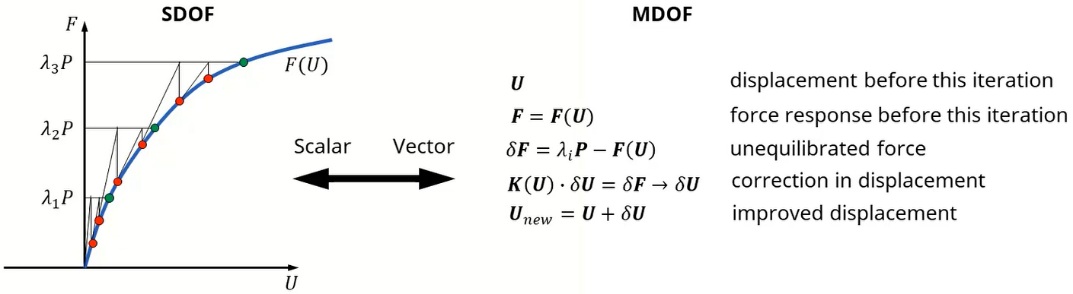To be able to visualize it, lets take a workaround.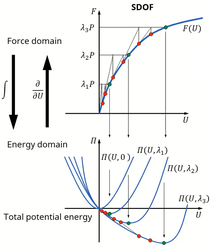Let us introduce the function of total potential energy. It is a scalar-valued function above the space of the state variables, which are the displacements. This function is connected to the equilibrium equations with an integral, differential relationship. In the view of the potential, different function belongs to each load factor and the solution point of the equilibrium equations at a certain load level is the minimum point of the related potential function.

These minimum points are marked with the green circles, while the red circles show the trial points of the iterations. Each of them is located on the curve related to the certain load increment. The reason why I introduced the potential function is that it remains scalar-valued for MDOF systems, as well.

Therefore we can visualize the 2 DOF case by drawing the potential function with an iso-surface view on the plane of the 2 displacement components.

In this view different map belongs to each load increment and the solution point of the equilibrium equations is the minimal point of this map. These are shown by the green circles, while the red circles show the trial points of the iterations. Each of them is located on the map related to the certain load increment. Comparing these maps with the SDOF curve, it can be seen that the iteration process in the MDOF case is not as simple as in the SDOF case. It seems like a rapsodic pathfinding procedure on a complex surface, rather than a monotonic approximation. Imagine it for 10.000 or 100.000 DOFs…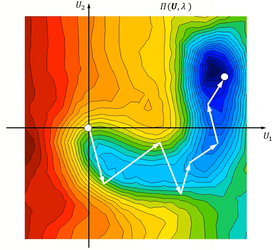Lets get a closer view of a map. For a large model with a lot of nonlinear elements, the hypersurface of the potential function can have a very complex geometry. In several cases, even more than one stable or unstable solution points may exist. Moreover, the solver cannot see the whole map. It can calculate the force response and the tangent stiffness only at the trial points. And it has to navigate on the basis of this few information.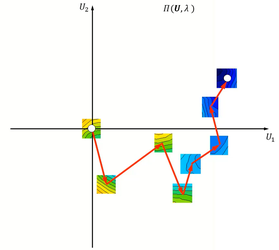The picture on the right shows quite expressively why the convergence of nonlinear analysis is so hard to achieve. Keep in mind, that the solver does not see the whole map, and does not know whether a trial point is close enough to the exact solution or not. It can only guess based on the fulfilment of some convergence criteria.